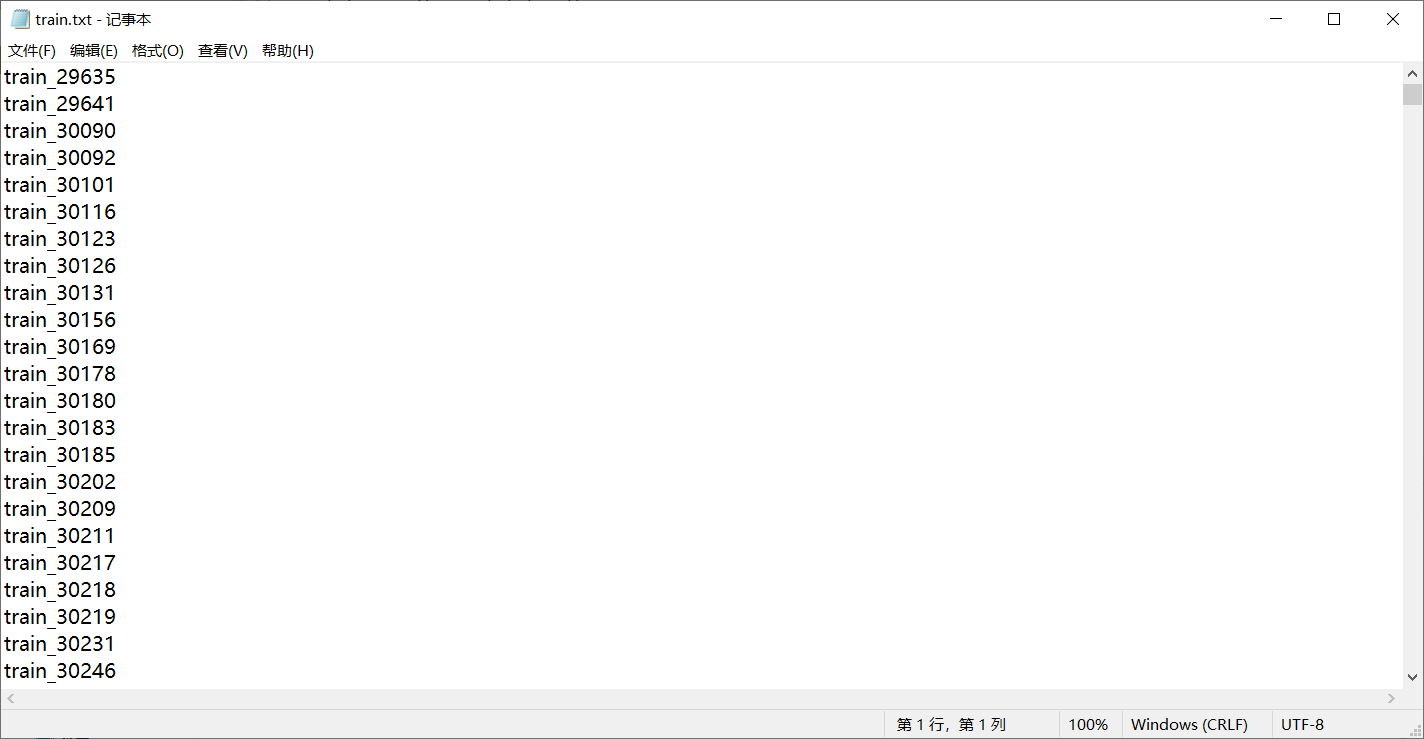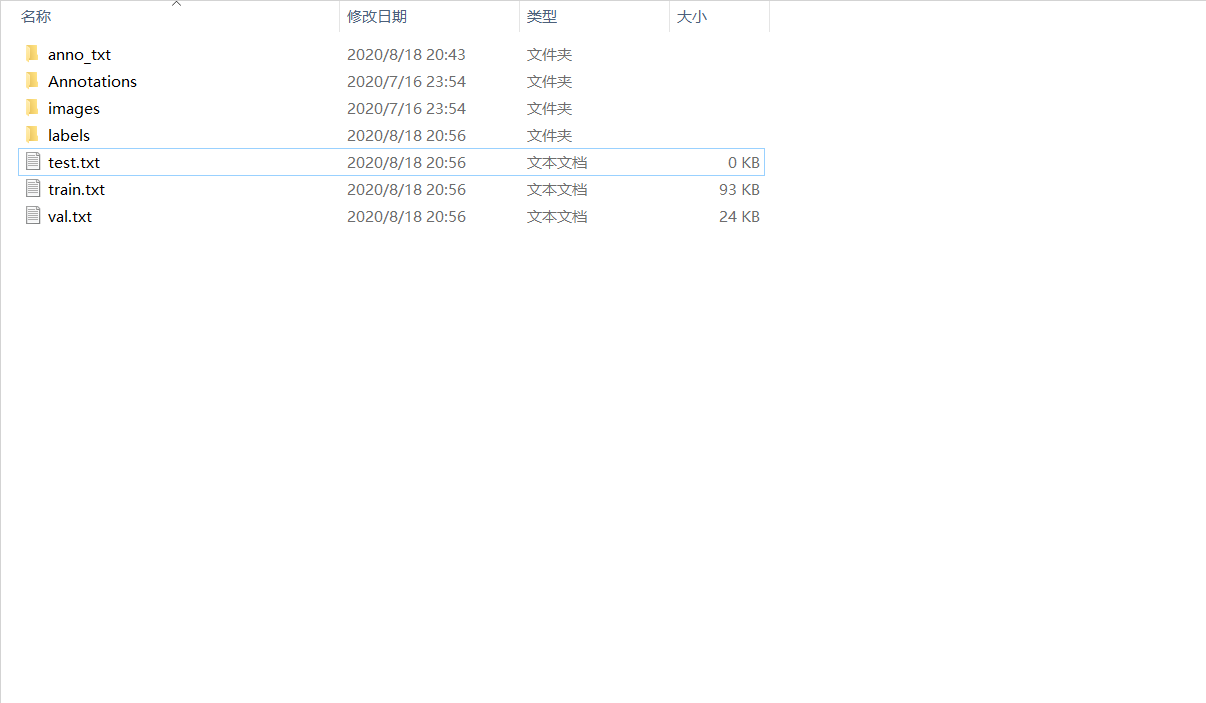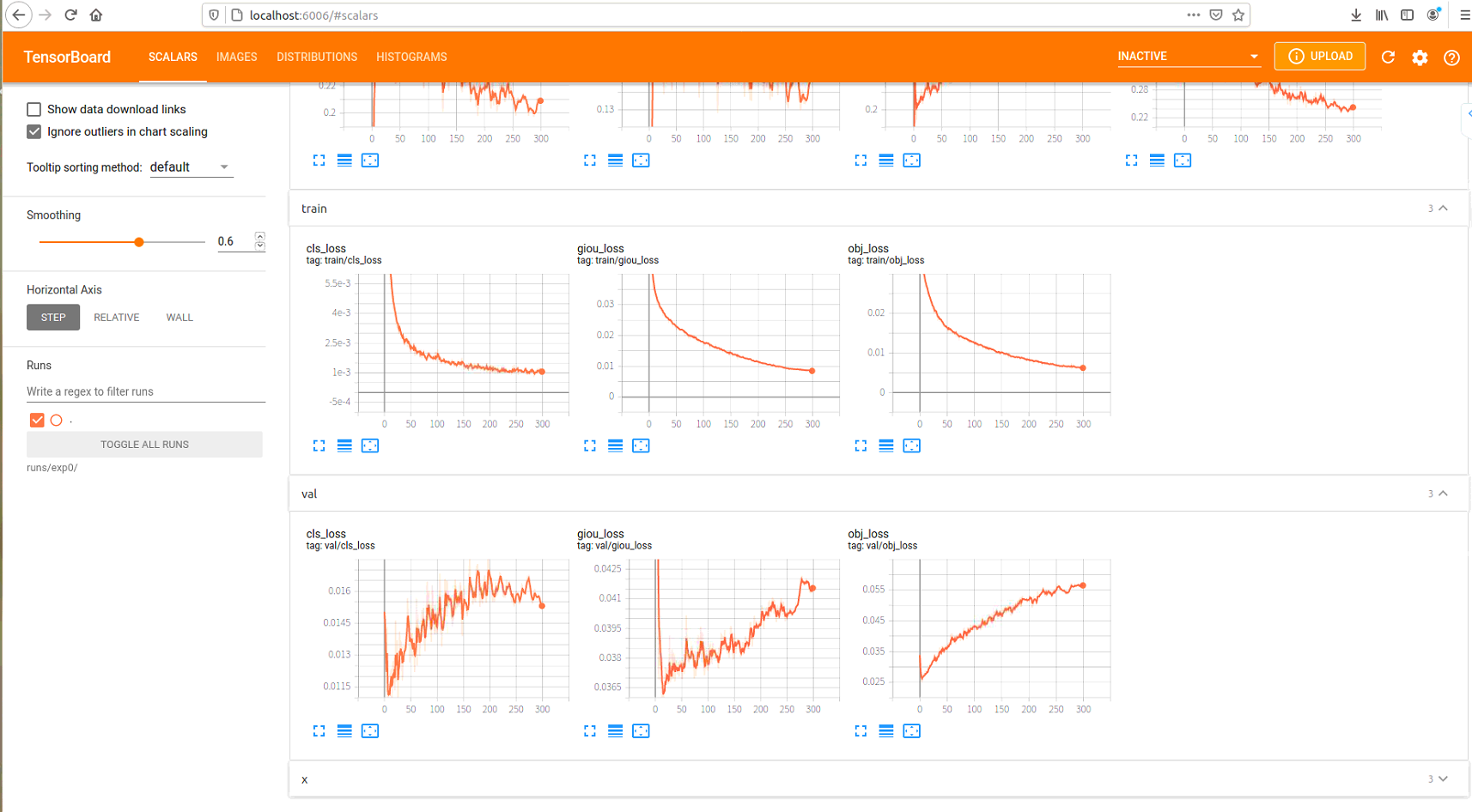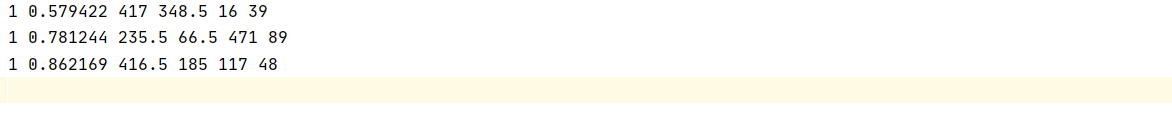# YOLOv5自定义数据集训练

## VOC数据集格式

- VOC
- JPEGImages
- 1.jpg
- 2.jpg
- ...
- Annotations
- 1.xml
- 2.xml
- ...
- ImageSets
- Main
- train.txt
- val.txt
- test.txt
- trainval.txt
- ...
- SegmentationClass
- SegmentationObject

<annotation>
<folder>down</folder> # 图片所处文件夹
<filename>1.jpg</filename> # 图片文件名及后缀
<path>./savePicture/train_29635.jpg</path> # 存放路径
<source>  #图源信息
<database>Unknown</database>
</source>
<size> # 图片尺寸和通道
<width>640</width>
<height>480</height>
<depth>3</depth>
</size>
<segmented>0</segmented>  #是否有分割label，0无1有
# 图像中包含的所有目标，一个目标一个object标签
<object>
<name>car</name>  # 目标类别
<pose>Unspecified</pose>  # 目标的姿态
<truncated>0</truncated>  # 目标是否被部分遮挡（>15%）
<difficult>0</difficult>  # 是否为难以辨识的目标， 需要结合背景才能判断出类别的物体
<bndbox>  # 目标边界框信息
<xmin>2</xmin>
<ymin>156</ymin>
<xmax>111</xmax>
<ymax>259</ymax>
</bndbox>
</object>
<object>
<name>multi_signs</name>
<editType />
<pose>Unspecified</pose>
<truncated>0</truncated>
<difficult>0</difficult>
<bndbox>
<xmin>81</xmin>
<ymin>98</ymin>
<xmax>154</xmax>
<ymax>243</ymax>
</bndbox>
</object>
</annotation>

## 自定义训练

### 数据集处理

- 根目录
- images
- Annotations

import os
import random
import argparse

parser = argparse.ArgumentParser()
parser.add_argument('--xml_path', type=str, help='input xml label path')
parser.add_argument('--txt_path', type=str, help='output txt label path')
opt = parser.parse_args()

trainval_percent = 1.0
train_percent = 0.8
xmlfilepath = opt.xml_path
txtsavepath = opt.txt_path
total_xml = os.listdir(xmlfilepath)
if not os.path.exists(txtsavepath):
os.makedirs(txtsavepath)

num = len(total_xml)
list_index = range(num)
tv = int(num * trainval_percent)
tr = int(tv * train_percent)
trainval = random.sample(list_index, tv)
train = random.sample(trainval, tr)

file_trainval = open(txtsavepath + '/trainval.txt', 'w')
file_test = open(txtsavepath + '/test.txt', 'w')
file_train = open(txtsavepath + '/train.txt', 'w')
file_val = open(txtsavepath + '/val.txt', 'w')

for i in list_index:
name = total_xml[i][:-4] + '\n'
if i in trainval:
file_trainval.write(name)
if i in train:
file_train.write(name)
else:
file_val.write(name)
else:
file_test.write(name)

file_trainval.close()
file_train.close()
file_val.close()
file_test.close()# -*- coding: utf-8 -*-

import xml.etree.ElementTree as ET
import os
from os import getcwd

sets = ['train', 'val', 'test']
classes = ['window_shielding', 'multi_signs', 'non_traffic_signs']
abs_path = os.getcwd()

def convert(size, box):
dw = 1. / (size)
dh = 1. / (size)
x = (box + box) / 2.0 - 1
y = (box + box) / 2.0 - 1
w = box - box
h = box - box
x = x * dw
w = w * dw
y = y * dh
h = h * dh
return x, y, w, h

def convert_annotation(image_id):
in_file = open('Annotations/%s.xml' % (image_id))
out_file = open('labels/%s.txt' % (image_id), 'w')
tree = ET.parse(in_file)
root = tree.getroot()
size = root.find('size')
w = int(size.find('width').text)
h = int(size.find('height').text)
for obj in root.iter('object'):
difficult = obj.find('difficult').text
cls = obj.find('name').text
if cls not in classes or int(difficult) == 1:
continue
cls_id = classes.index(cls)
xmlbox = obj.find('bndbox')
b = (float(xmlbox.find('xmin').text), float(xmlbox.find('xmax').text), float(xmlbox.find('ymin').text),
float(xmlbox.find('ymax').text))
b1, b2, b3, b4 = b
# 标注越界修正
if b2 > w:
b2 = w
if b4 > h:
b4 = h
b = (b1, b2, b3, b4)
bb = convert((w, h), b)
out_file.write(str(cls_id) + " " + " ".join([str(a) for a in bb]) + '\n')

wd = getcwd()
for image_set in sets:
if not os.path.exists('labels/'):
os.makedirs('labels/')
list_file = open('%s.txt' % (image_set), 'w')
for image_id in image_ids:
list_file.write(abs_path + '/images/%s.jpg\n' % (image_id))
convert_annotation(image_id)
list_file.close()### 配置文件

train: dataset/train/train.txt
val: dataset/train/val.txt

# number of classes
nc: 3

# class names
names: ['window_shielding', 'multi_signs', 'non_traffic_sign']

### 模型训练

python train.py --img 640 --batch 8 --epoch 300 --data ./data/ads.yaml --cfg ./models/yolov5x.yaml --weights weights/yolov5x.pt --device '0'### 模型测试

python test.py  --data ./data/ads.yaml --weights ./runs/exp0/weights/best.pt --augment

Class  Images  Targets   P       R      mAP@.5    mAP@.5:.95
all    400      970    0.376    0.441     0.35       0.235

Class  Images  Targets    P      R      mAP@.5    mAP@.5:.95
all     400     970     0.272   0.532   0.366        0.24

### 模型推理

python detect.py --weights runs/exp0/weights/best.pt --source ./dataset/test/ --device 0 --save-txt目录